# The Verilog Hardware Description Language GUIDELINES n How

• Slides: 64The Verilog Hardware Description LanguageGUIDELINES n How to write HDL code:GUIDELINES n How NOT to write HDL code:Think Hardware NOT Software n Poorly written HDL code will either be: – Unsynthesizable – Functionally incorrect – Lead to poor performance/area/power results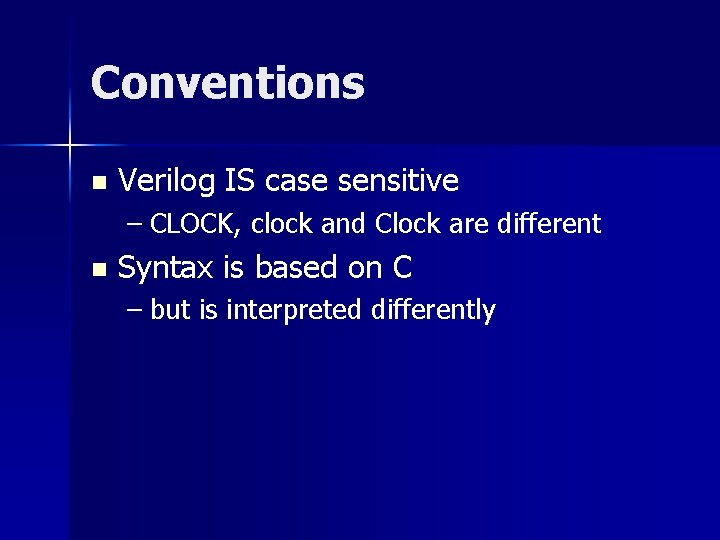Conventions n Verilog IS case sensitive – CLOCK, clock and Clock are different n Syntax is based on C – but is interpreted differently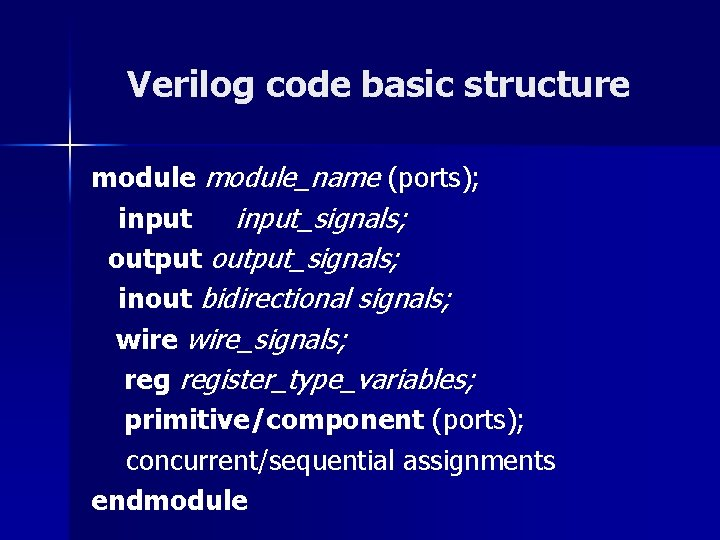Verilog code basic structure module_name (ports); input_signals; output_signals; inout bidirectional signals; wire_signals; register_type_variables; primitive/component (ports); concurrent/sequential assignments endmodule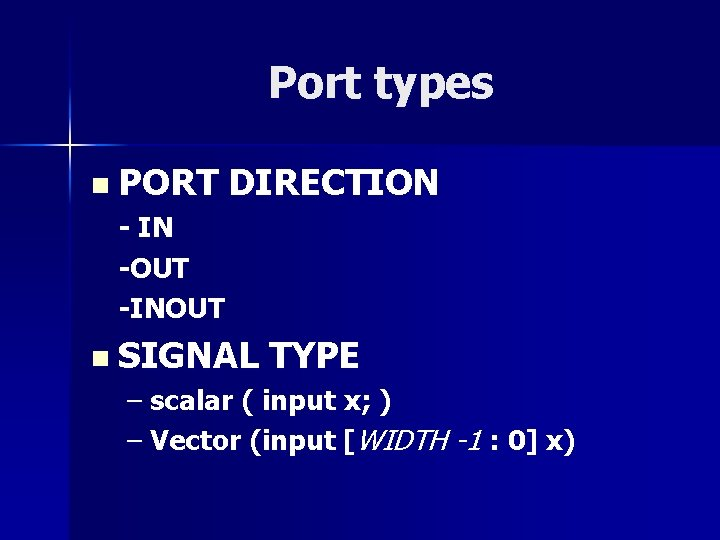Port types n PORT DIRECTION - IN -OUT -INOUT n SIGNAL TYPE – scalar ( input x; ) – Vector (input [WIDTH -1 : 0] x)Module port declaration example (1/2) module and_gate (o, i 1, i 2); output o; input i 1; input i 2; endmoduleModule port declaration example (2/2) module adder (carry, sum, i 1, i 2); output carry; output [3: 0] sum; input [3: 0] i 1, i 2; endmoduleExampleVerilog Primitives Verilog primitives are models of common combinational logic gates n Their functionality is built into the language and can be instantiated in designs directly n The output port of a primitive must be first in the list of ports n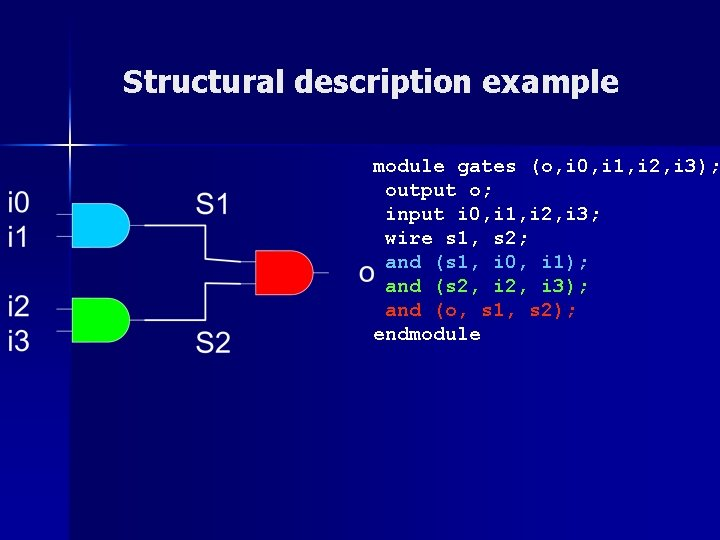Structural description example module gates (o, i 0, i 1, i 2, i 3); output o; input i 0, i 1, i 2, i 3; wire s 1, s 2; and (s 1, i 0, i 1); and (s 2, i 3); and (o, s 1, s 2); endmoduleVerilog operators • Arithmetic +, - , *, /, % • Bitwise & AND | OR ~ NOT ^ XOR ~^ XNOR Relational =, <, >, <=, >= • Synthesizable Non-synthesizable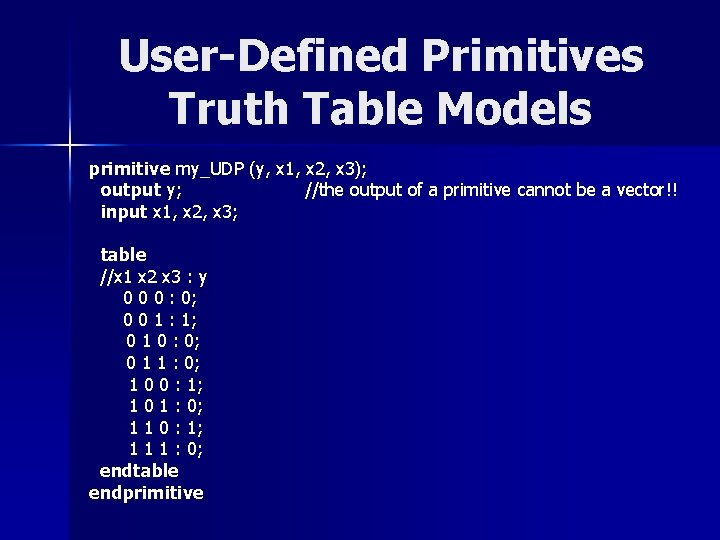User-Defined Primitives Truth Table Models primitive my_UDP (y, x 1, x 2, x 3); output y; //the output of a primitive cannot be a vector!! input x 1, x 2, x 3; table //x 1 x 2 x 3 : y 0 0 0 : 0; 0 0 1 : 1; 0 1 0 : 0; 0 1 1 : 0; 1 0 0 : 1; 1 0 1 : 0; 1 1 0 : 1; 1 1 1 : 0; endtable endprimitive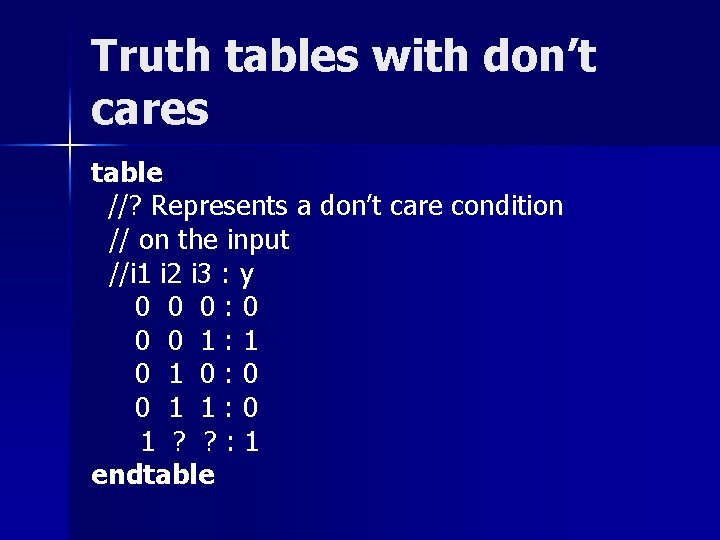Truth tables with don’t cares table //? Represents a don’t care condition // on the input //i 1 i 2 i 3 : y 0 0 0: 0 0 0 1: 1 0: 0 0 1 1: 0 1 ? ? : 1 endtablevariable assignment variable_name = value; //blocking assignment variable_name <= value; //non-blocking assignment output a; output [6: 0] b; reg [3: 0] c; wire [2: 0] d; Correct a = 1; b <= 7’b 0101001; b = 0; c <= 0; d <= {b , c}; b <= {c, d}; b[5: 2] <= c; Examples Incorrect a <= 3; b = 9’b 000011011; d <= 0;Propagation delay Used to assign variables or primitives with delay, modeling circuit behaviour n # 5 and (s, i 0, i 1); -- 5 ns and gate delay n #5 assign s = i 0 & i 1; -- 5 ns and gate delay n #1 assign a = b; --1 ns wire delay Not synthesizable, is ignored by synthesis tools Useful in testbenches for creating input signal waveforms n always #20 clk = ~clk -- 40 ns clock period n #0 rst_n = 0; n #10 rst_n = 1;Concurrent statements – delta time b = ~ a; (a = 1, b = 1, c =0) c = a ^ b; Time 0 δ 2δ a 1 1 1 b 1 0 0 c 0 0 1Continuous assignment n Multiple driver error assign c = a & b; …. assign c = d | e;Combinational circuit description module gates (d, a, c); output d; input a, c; //wire b; assign d = c ^ (~a); // assign b = ~a; // assign d = c ^ b; endmoduleArithmetic unit description (full-adder) module add 1 (cout, sum, a, b, cin); input a, b; input cin; output sum; output cout; assign {cout, sum} = a + b + cin; endmoduleExample Describe a 5 -bit multiplier in Verilog.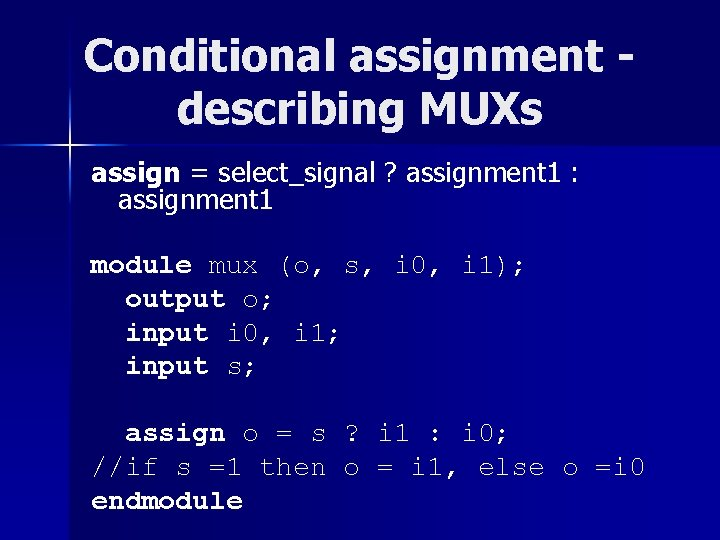Conditional assignment describing MUXs assign = select_signal ? assignment 1 : assignment 1 module mux (o, s, i 0, i 1); output o; input i 0, i 1; input s; assign o = s ? i 1 : i 0; //if s =1 then o = i 1, else o =i 0 endmoduleCyclic behavior • • • Statements in cyclic behavior execute sequentially Can be used to describe either combinational circuits (optional) or sequential circuits (only way) Signals assigned in always blocks must be of type reg always & (sensitivity list) begin sequential statements end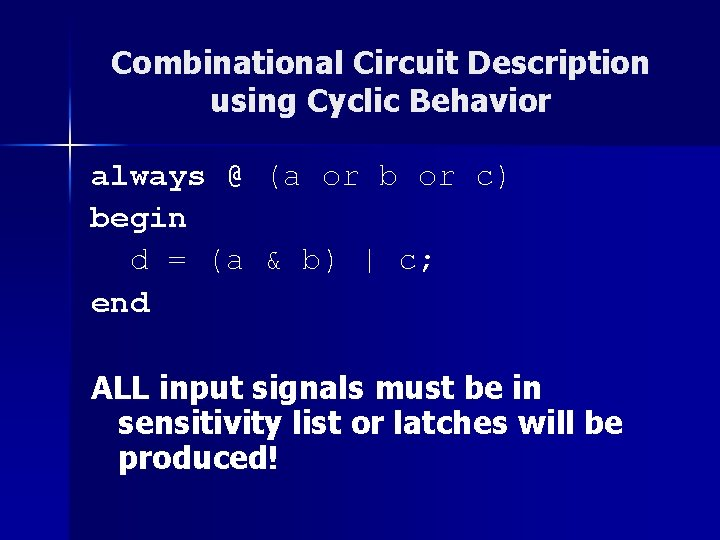Combinational Circuit Description using Cyclic Behavior always @ (a or b or c) begin d = (a & b) | c; end ALL input signals must be in sensitivity list or latches will be produced!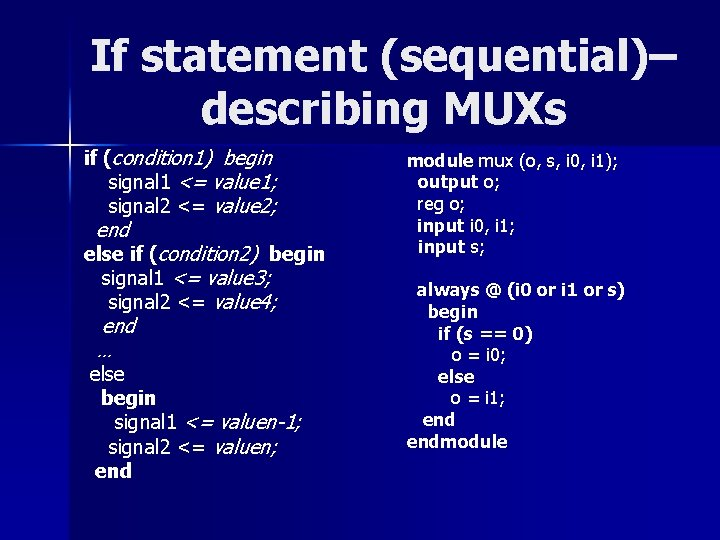If statement (sequential)– describing MUXs if (condition 1) begin signal 1 <= value 1; signal 2 <= value 2; end else if (condition 2) begin signal 1 <= value 3; signal 2 <= value 4; end … else begin signal 1 <= valuen-1; signal 2 <= valuen; end module mux (o, s, i 0, i 1); output o; reg o; input i 0, i 1; input s; always @ (i 0 or i 1 or s) begin if (s == 0) o = i 0; else o = i 1; endmodule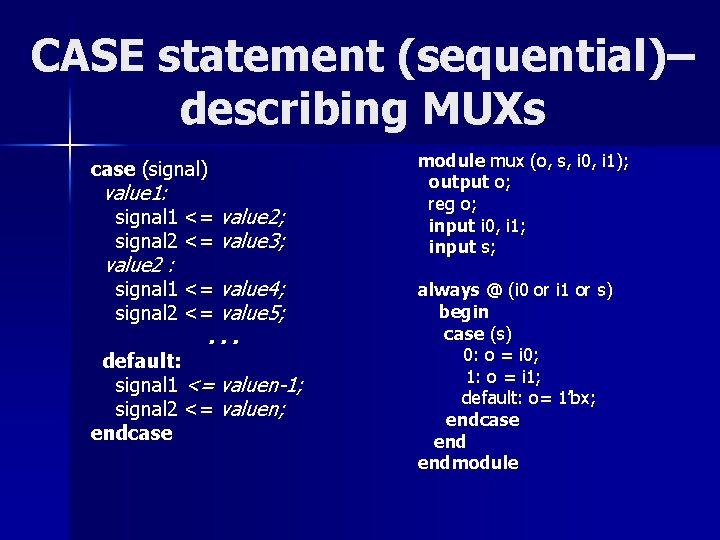CASE statement (sequential)– describing MUXs case (signal) value 1: signal 1 <= value 2; signal 2 <= value 3; value 2 : signal 1 <= value 4; signal 2 <= value 5; . . . default: signal 1 <= valuen-1; signal 2 <= valuen; endcase module mux (o, s, i 0, i 1); output o; reg o; input i 0, i 1; input s; always @ (i 0 or i 1 or s) begin case (s) 0: o = i 0; 1: o = i 1; default: o= 1’bx; endcase endmoduleExample n Describe a 3 -bit 4 -to-1 MUX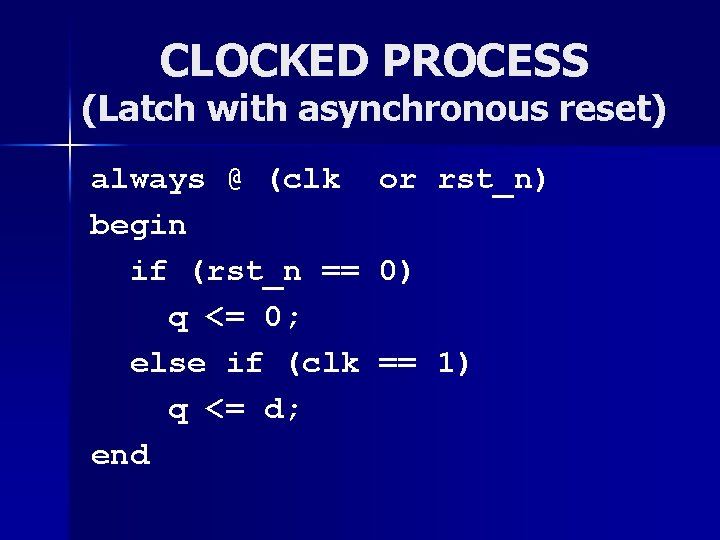CLOCKED PROCESS (Latch with asynchronous reset) always @ (clk begin if (rst_n == q <= 0; else if (clk q <= d; end or rst_n) 0) == 1)CLOCKED PROCESS (Latch with synchronous reset) always @ begin if (clk q <= else if q <= end (clk or rst_n) == 1) d; (rst_n == 0) 0;CLOCKED PROCESS (Flip-flop with asynchronous reset) always @(posedge clk or negedge rst_n) begin if (rst_n == 0) q <= 0; else q <= d; endCLOCKED PROCESS (Flip-flop with synchronous reset) always @(posedge begin if (rst_n == q <= 0; else q <= d; end clk) 0)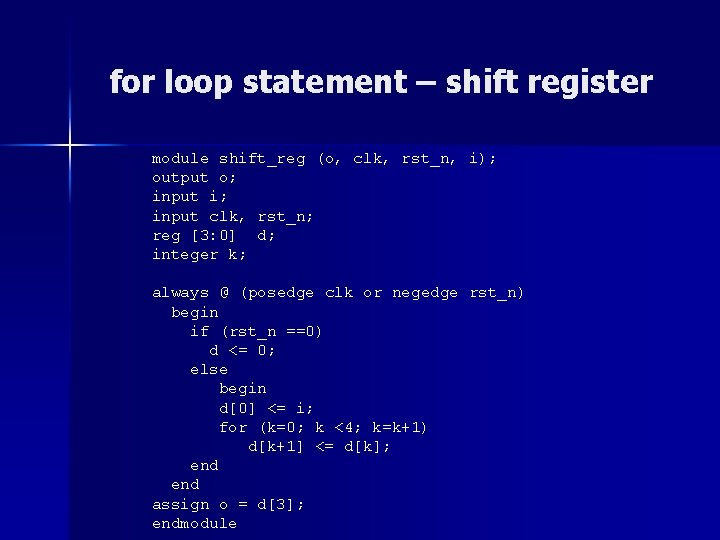for loop statement – shift register module shift_reg (o, clk, rst_n, i); output o; input i; input clk, rst_n; reg [3: 0] d; integer k; always @ (posedge clk or negedge rst_n) begin if (rst_n ==0) d <= 0; else begin d <= i; for (k=0; k <4; k=k+1) d[k+1] <= d[k]; end assign o = d; endmoduleCLOCKED VS COMBINATIONAL PROCESS (1/2) always @ (a or b or c) begin case (c) 0: q = a; 1: q = b; default: q= 1’bx; endcase end always @ (posedge clk or negedge rst_n) begin if (rst_n == 0) q <= 0; else case (c) 0: q = a; 1: q = b; default: q= 1’bx; endcase endCLOCKED VS COMBINATIONAL PROCESS (2/2) always @ (a or b or c) begin d = (a & b) | c; end always @ (posedge clk) begin if (rst_n == 0) d <= 0; else d <= (a & b) | c; endEXAMPLE n DESCIBE A BINARY UP/DOWN COUNTER WITH ENABLE THAT COUNTS UPTO 12 AND THEN STARTS AGAIN FROM ZEROTESTBENCH `timescale 1 ns / 100 ps module testbench_name (); reg …. ; //declaration of register variables for DUT inputs wire …; //declaration of wires for DUT outputs DUT_name(DUT ports); initial \$monitor(); //signals to be monitored (optional) initial begin #100 \$finish; //end simulation end initial begin clk = 1’b 0; #10 a = 1; … end //initialize clk always # 50 clk = ~clk; //50 ns clk period (if there is a clock) endmoduleTESTBENCH EXAMPLE `timescale 1 ns / 100 ps module mux_tb (); reg i 0, i 1, s; wire o; initial begin //stimulus pattern #10 i 0 = 0; i 1=0; s=0; #10 i 0=1; #10 i 0 = 0; i 1=1; #10 i 0=0; i 1= 0; s=1; mux M 1 (o, s, i 0, i 1); #10 i 0=1; #10 i 0 = 0; i 1=1; initial begin #10 i 0=1; #100 \$finish; //end simulation end endmodule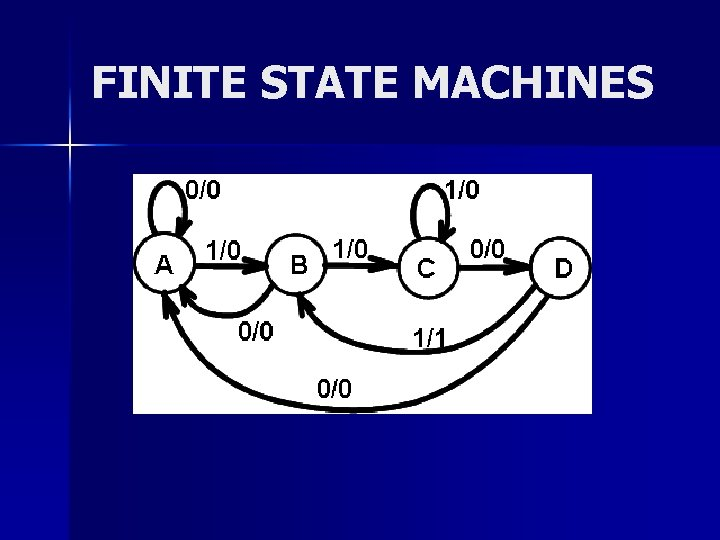FINITE STATE MACHINES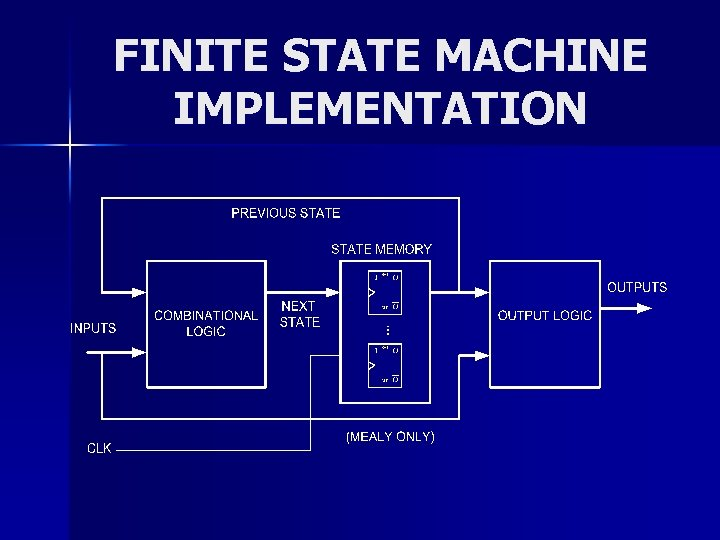FINITE STATE MACHINE IMPLEMENTATIONMealy machines (1/5) module fsm (y, clk, rst_n, x); output y; input clk, rst_n, x; reg [1: 0] state_pr, state_nx; reg y; parameter a = 0, b = 1, c = 2, d = 3, dont_care_state = 2’bx, dont_care_out = 1’bx;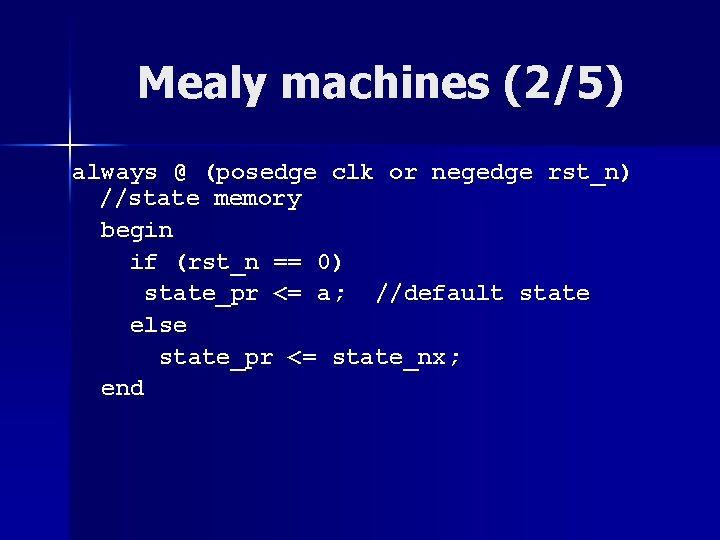Mealy machines (2/5) always @ (posedge clk or negedge rst_n) //state memory begin if (rst_n == 0) state_pr <= a; //default state else state_pr <= state_nx; end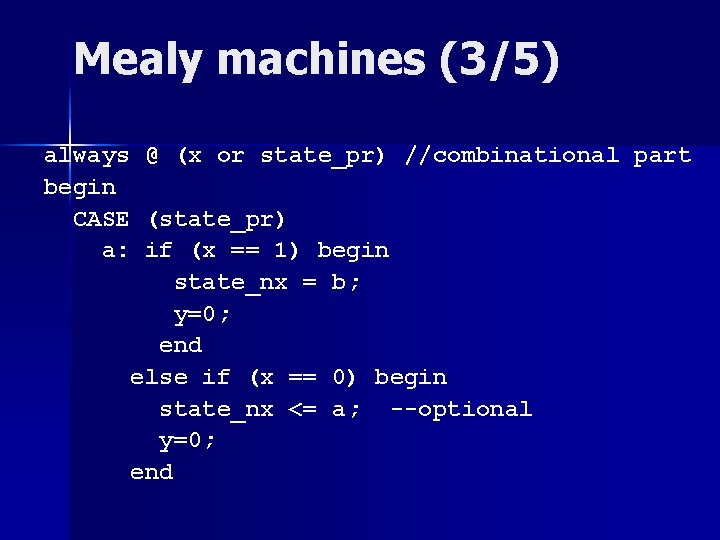Mealy machines (3/5) always @ (x or state_pr) //combinational part begin CASE (state_pr) a: if (x == 1) begin state_nx = b; y=0; end else if (x == 0) begin state_nx <= a; --optional y=0; end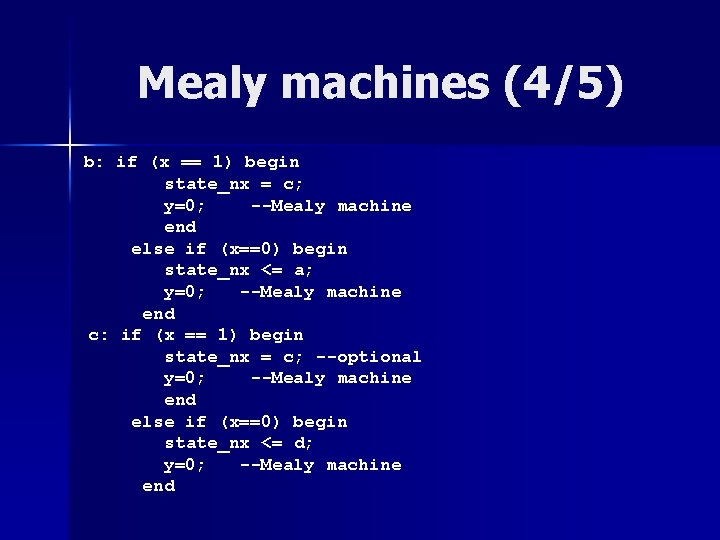Mealy machines (4/5) b: if (x == 1) begin state_nx = c; y=0; --Mealy machine end else if (x==0) begin state_nx <= a; y=0; --Mealy machine end c: if (x == 1) begin state_nx = c; --optional y=0; --Mealy machine end else if (x==0) begin state_nx <= d; y=0; --Mealy machine endMealy machines (5/5) d: if (x == 1) begin state_nx = b; y=1; --Mealy machine end else if (x==0) begin state_nx <= a; y=0; --Mealy machine end default: begin state_nx <= dont_care_state; y <= dont_care_out; endcase endmoduleMoore machines always @ (x or state_pr) --combinational part begin CASE (state_pr) s 0: y = <value>; --Moore machine if (a == 1) state_nx = s 1; else state_nx = s 0; --optional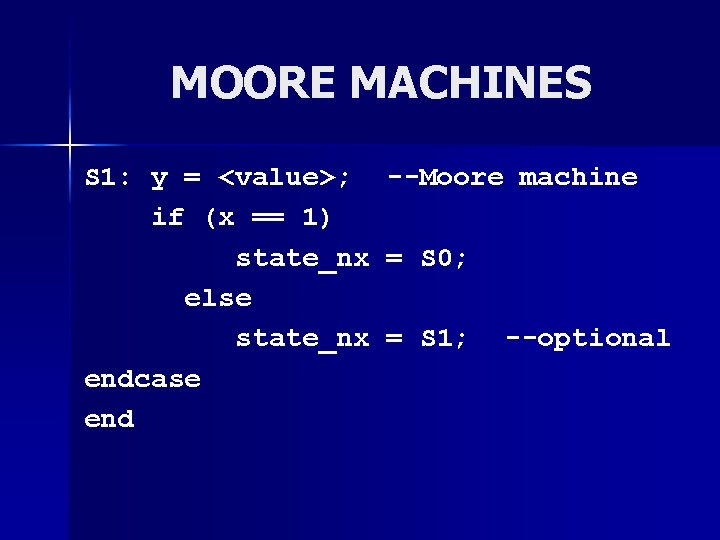MOORE MACHINES S 1: y = <value>; --Moore machine if (x == 1) state_nx = S 0; else state_nx = S 1; --optional endcase end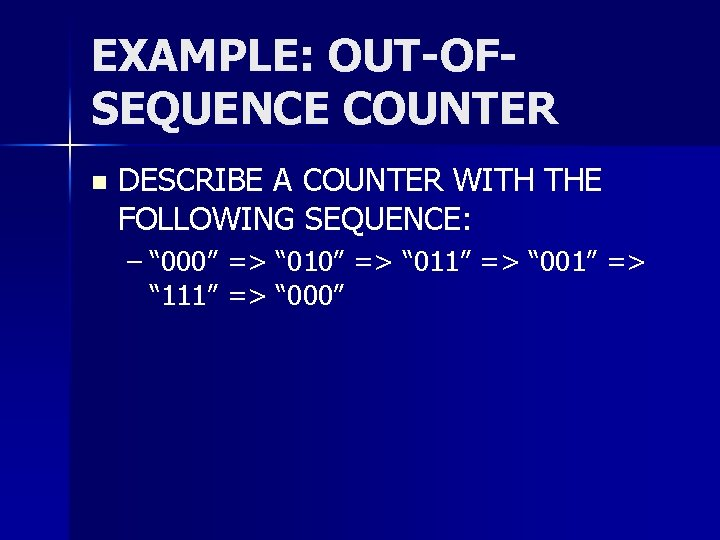EXAMPLE: OUT-OFSEQUENCE COUNTER n DESCRIBE A COUNTER WITH THE FOLLOWING SEQUENCE: – “ 000” => “ 011” => “ 001” => “ 111” => “ 000”ROM description (1/2) module rominfr (data, en, addr); output [3: 0] data; input en; input [4: 0] addr; reg [3: 0] ROM [31: 0]; assign data = ROM[addr];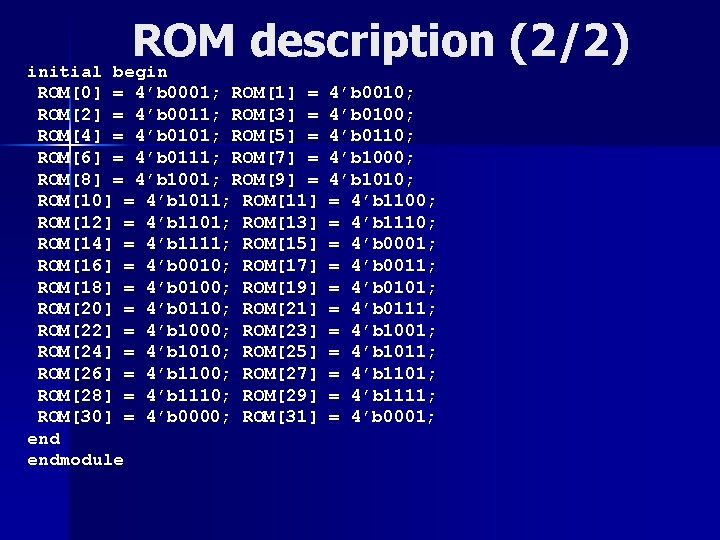ROM description (2/2) initial begin ROM = 4’b 0001; ROM = ROM = 4’b 0011; ROM = ROM = 4’b 0101; ROM = ROM = 4’b 0111; ROM = ROM = 4’b 1001; ROM = ROM = 4’b 1011; ROM ROM = 4’b 1101; ROM ROM = 4’b 1111; ROM ROM = 4’b 0010; ROM ROM = 4’b 0100; ROM ROM = 4’b 0110; ROM ROM = 4’b 1000; ROM ROM = 4’b 1010; ROM ROM = 4’b 1100; ROM ROM = 4’b 1110; ROM ROM = 4’b 0000; ROM endmodule 4’b 0010; 4’b 0100; 4’b 0110; 4’b 1000; 4’b 1010; = 4’b 1100; = 4’b 1110; = 4’b 0001; = 4’b 0011; = 4’b 0101; = 4’b 0111; = 4’b 1001; = 4’b 1011; = 4’b 1101; = 4’b 1111; = 4’b 0001;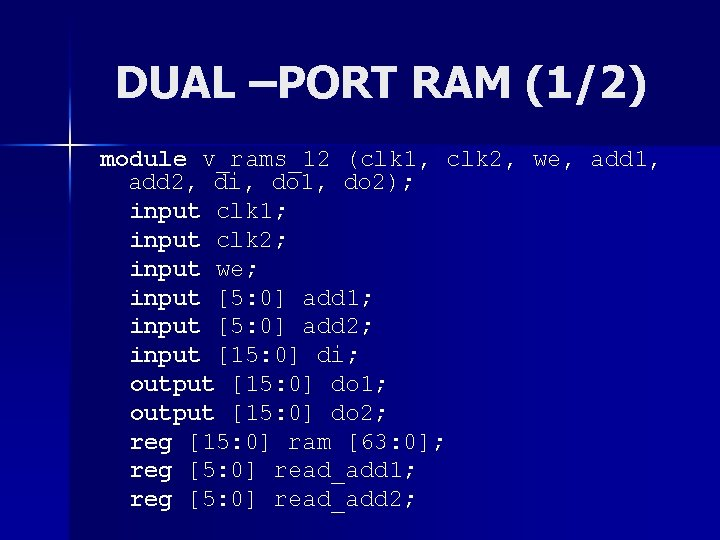DUAL –PORT RAM (1/2) module v_rams_12 (clk 1, clk 2, we, add 1, add 2, di, do 1, do 2); input clk 1; input clk 2; input we; input [5: 0] add 1; input [5: 0] add 2; input [15: 0] di; output [15: 0] do 1; output [15: 0] do 2; reg [15: 0] ram [63: 0]; reg [5: 0] read_add 1; reg [5: 0] read_add 2;Include files `include "timing. vh" module counter 1(count, reset, clk) ; output [7: 0] count; input reset, clk; reg [7: 0] count; always @(posedge clk or posedge reset) if(reset) count <= #(`REG_DELAY) 8'b 0; else count <= #(`REG_DELAY) count + 8 'b 1; . . . n //contents of ”timing. vh” file `timescale Ins/Ins `define REG_DELAY 1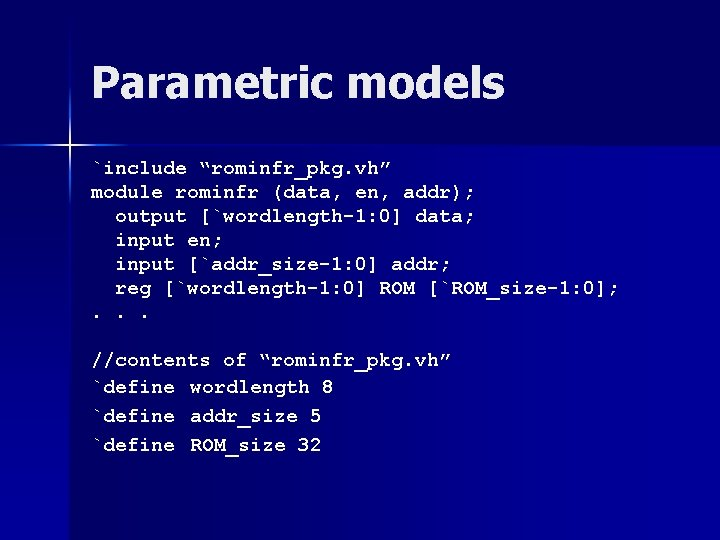Parametric models `include “rominfr_pkg. vh” module rominfr (data, en, addr); output [`wordlength-1: 0] data; input en; input [`addr_size-1: 0] addr; reg [`wordlength-1: 0] ROM [`ROM_size-1: 0]; . . . //contents of “rominfr_pkg. vh” `define wordlength 8 `define addr_size 5 `define ROM_size 32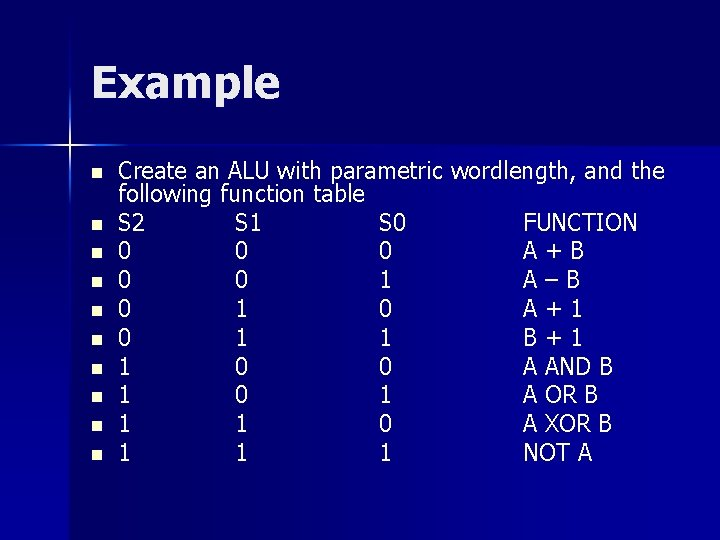Example n n n n n Create an ALU with parametric wordlength, and the following function table S 2 S 1 S 0 FUNCTION 0 0 0 A+B 0 0 1 A–B 0 1 0 A+1 0 1 1 B+1 1 0 0 A AND B 1 0 1 A OR B 1 1 0 A XOR B 1 1 1 NOT ACommon Verilog Pitfalls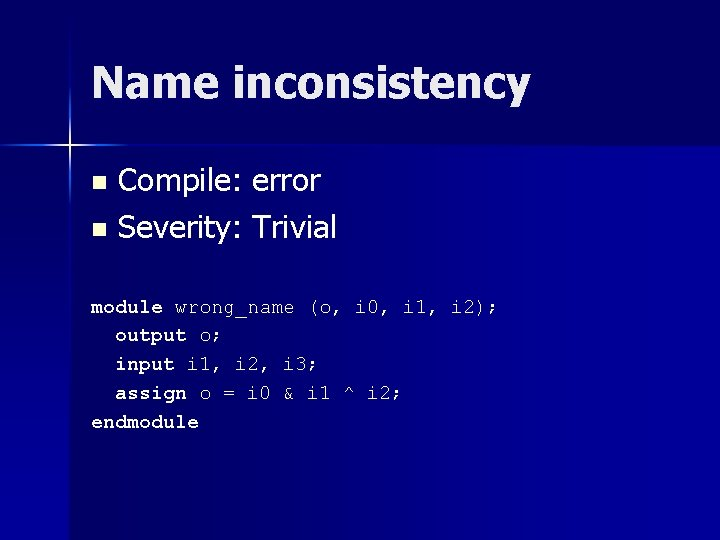Name inconsistency Compile: error n Severity: Trivial n module wrong_name (o, i 0, i 1, i 2); output o; input i 1, i 2, i 3; assign o = i 0 & i 1 ^ i 2; endmoduleMultiple unconditional concurrent assignments n n n Simulation: ‘X’ value Synthesis: ERROR: signal is multiply driven Severity: Serious module … assign x = a & b; . . . assign x = b ^ c; always @ (b or c) begin x <= b | c; end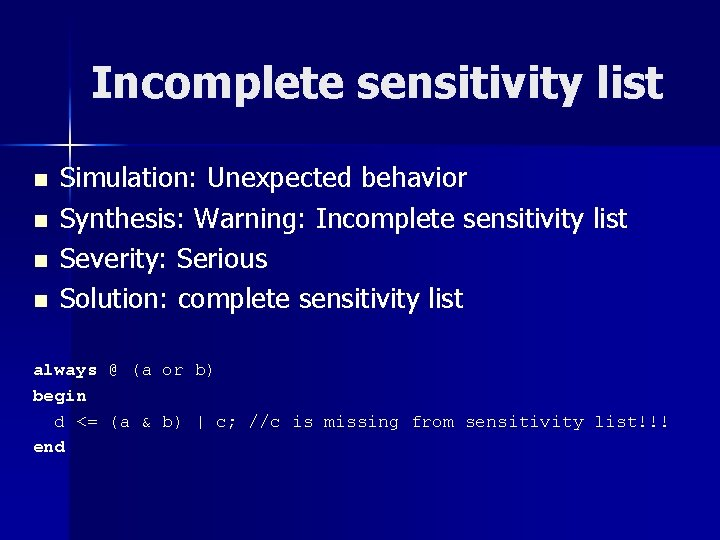Incomplete sensitivity list n n Simulation: Unexpected behavior Synthesis: Warning: Incomplete sensitivity list Severity: Serious Solution: complete sensitivity list always @ (a or b) begin d <= (a & b) | c; //c is missing from sensitivity list!!! end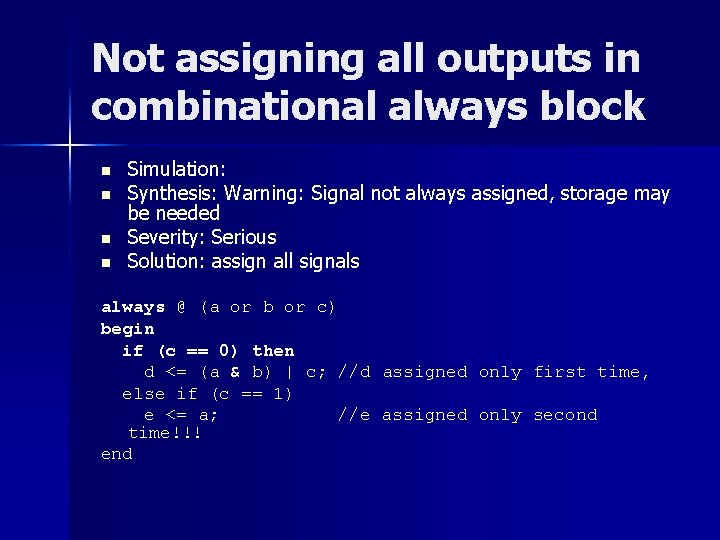Not assigning all outputs in combinational always block n n Simulation: Synthesis: Warning: Signal not always assigned, storage may be needed Severity: Serious Solution: assign all signals always @ (a or b or c) begin if (c == 0) then d <= (a & b) | c; //d assigned only first time, else if (c == 1) e <= a; //e assigned only second time!!! end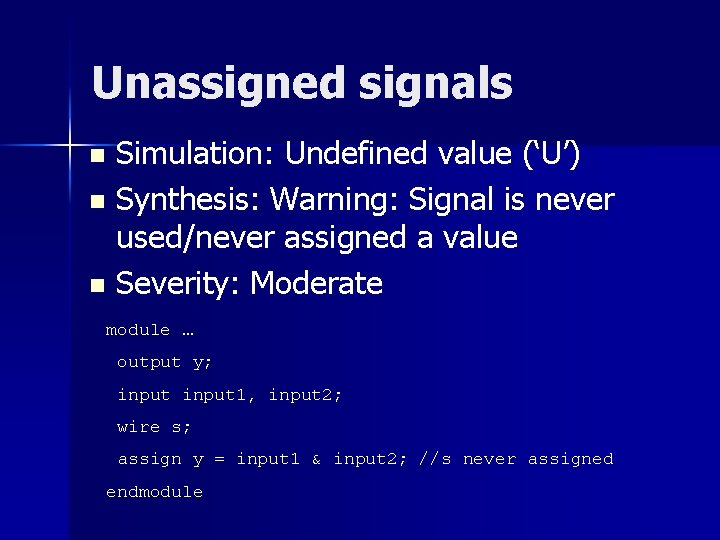Unassigned signals Simulation: Undefined value (‘U’) n Synthesis: Warning: Signal is never used/never assigned a value n Severity: Moderate n module … output y; input 1, input 2; wire s; assign y = input 1 & input 2; //s never assigned endmodule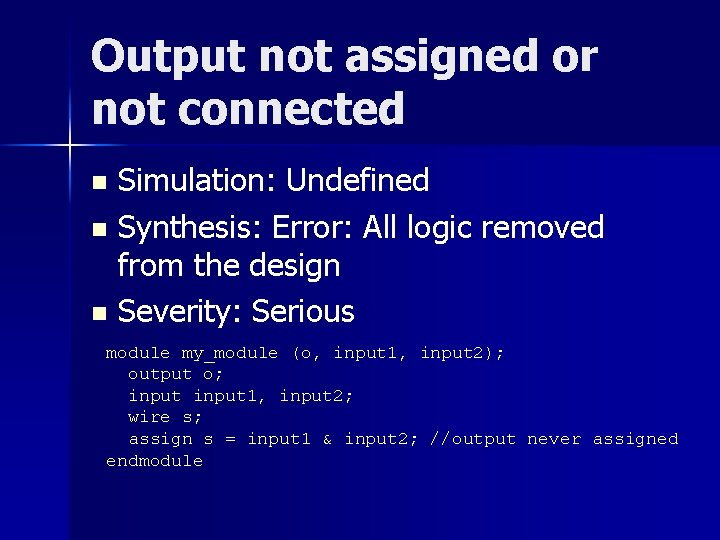Output not assigned or not connected Simulation: Undefined n Synthesis: Error: All logic removed from the design n Severity: Serious n module my_module (o, input 1, input 2); output o; input 1, input 2; wire s; assign s = input 1 & input 2; //output never assigned endmodule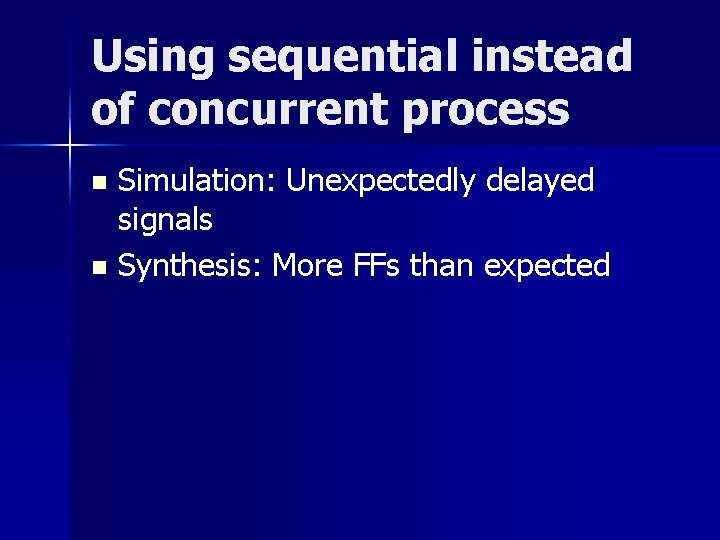Using sequential instead of concurrent process Simulation: Unexpectedly delayed signals n Synthesis: More FFs than expected nConfusing reg with wire module my_module (o, input 1, input 2); output o; input 1, input 2; assign o = input 1 & input 2; //output is wire by default endmodule my_module (o, input 1, input 2); output o; reg o; input 1, input 2; always @ (input 1 or input 2) //using always block o = input 1 & input 2; //output must be reg endmodule